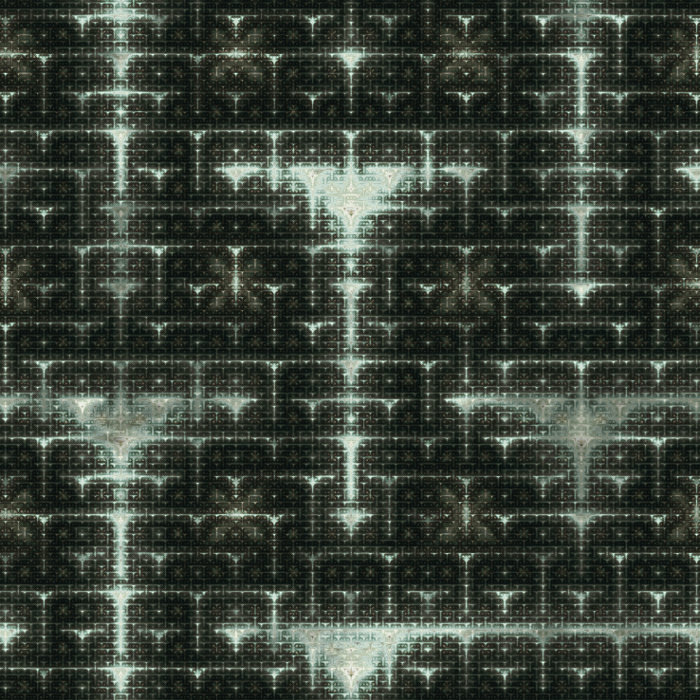### 20100714-1

2010, digital image and unique archival pigment print

The zoomable versions of the works are currently unavailable.

Back to the index

Septembre 12th 2010

## Truchet patterns II

So here is the follow up on Truchet patterns promised a long time ago (in the previous post... :S).

Recall that to draw a Truchet pattern, you have to make random choices. Truchet patterns are constructed from a tiling (usually a square one) by choosing randomly a decoration on each tile. If the decorations are carefully designed, a continuous non-periodic pattern emerges.

The point I would like to make in this post is that you can get very interesting patterns if you do not make the choice of decoration randomly. Let us see first how the random choice is implemented. As we need one decoration for each tile, we need one random number per tile. One way to get one random number per tile is to devise a complicated real-valued function on the plane which varies wildly, and evaluate it at the center of each tile.

All the patterns implemented in my algorithms are also real-valued functions on the plane. So what if, instead of using a complicated function to get random numbers for each tile, we use simply another pattern (that we will call the choice pattern in the following) and get not-so-random numbers for each tile? Then some very interesting patterns appear. Let us first have a look at a standard Truchet pattern, for which we used random choices of the decorations: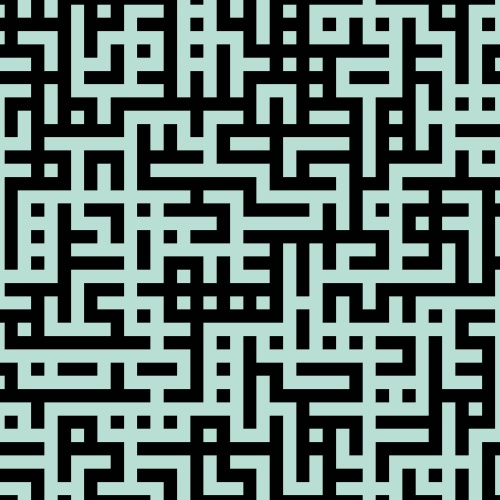Now suppose we used a very dumb choice pattern like the following one to pick up the decorations: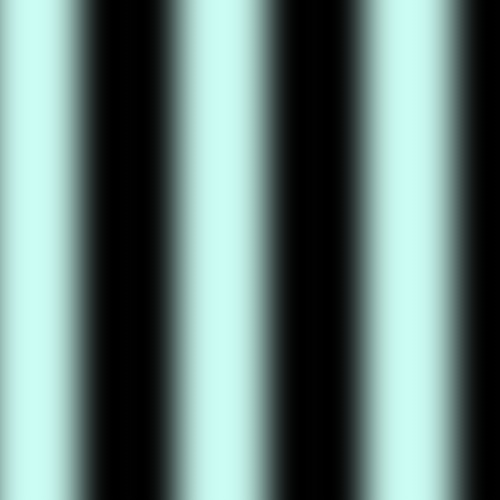We get the following Truchet pattern: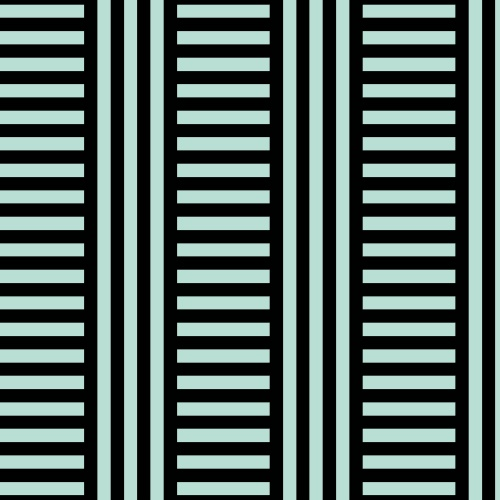Admittedly, it is not yet very interesting. But look what happens if we reduce the separation of the vertical lines of the choice pattern, so that it is of the same magnitude as the tiles of the Truchet pattern: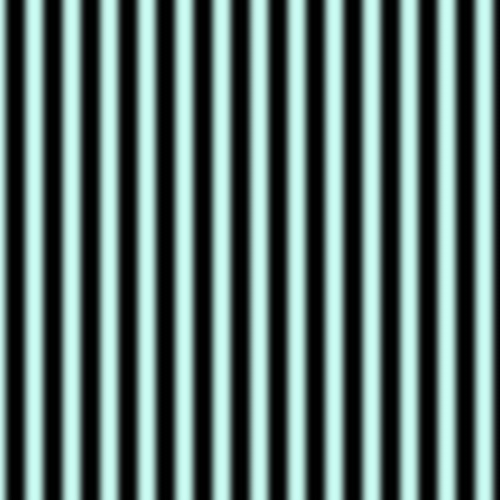We get the following surprising pattern: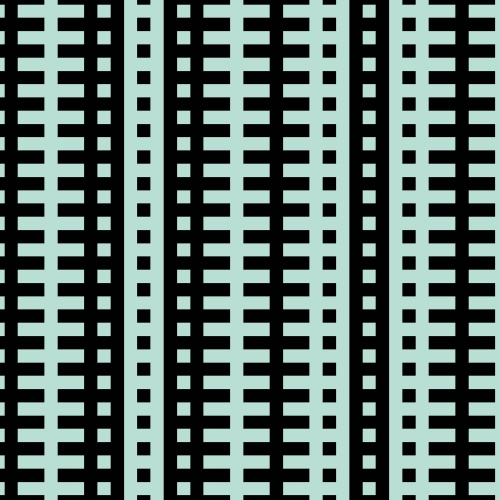Changing the separation of the lines in the choice pattern gives several other nice patterns: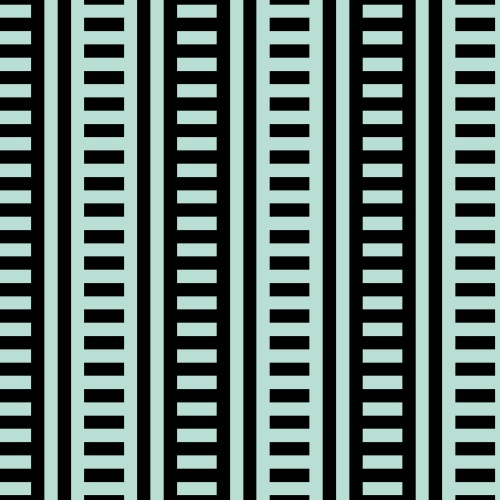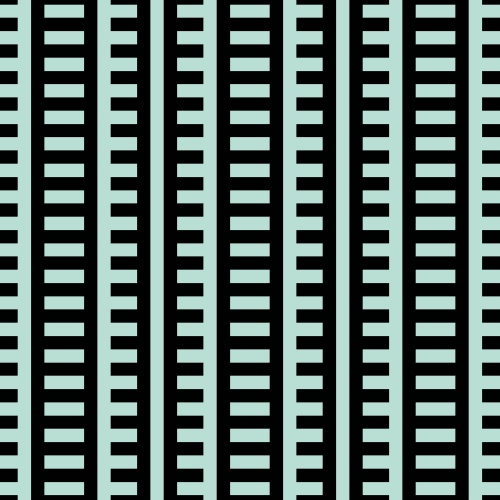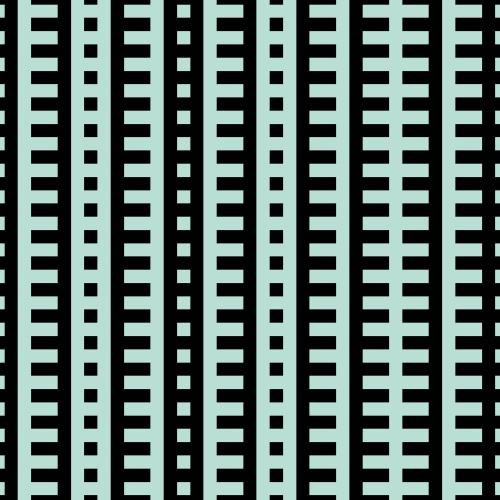This was just using an utterly simple choice pattern: vertical lines. The next simplest choice is to use a square gird as a choice pattern: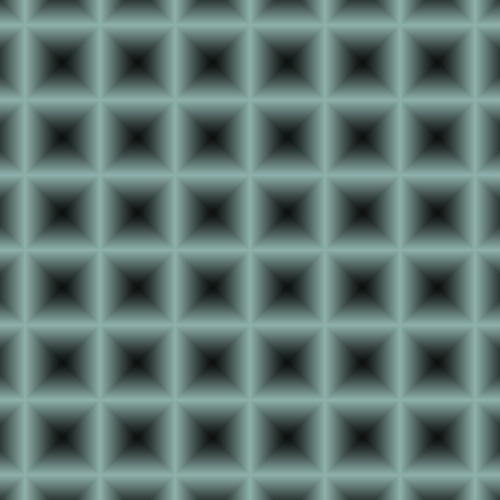which yields: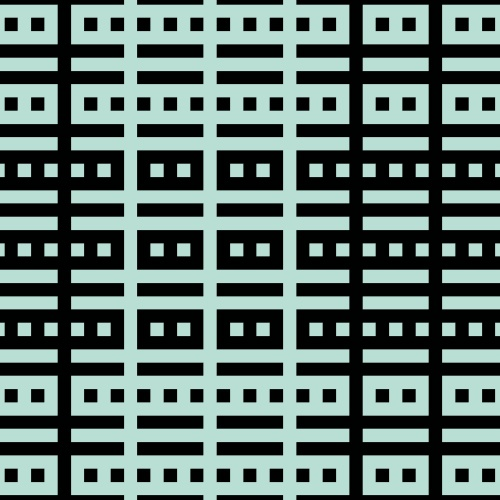Again, variating the width and height of the gird gives a swarm of non-random Truchet pattern. Note that unless the height and the width are carefully tuned (to rational multiples of the height and width of the tiles), the patterns obtained are not periodic. The periodicity breaking can sometimes be subtle, or invisible on the portion of the pattern rendered on the picture. Of course, this technique applies not only to Truchet patterns, but to any pattern that requires random numbers that can be associated to specific places of the plane (like the center of each tile in the case of the Truchet pattern).

Finally, here are two works created from such non-random Truchet patterns. You can find more of them in this series.

20080720

20090718-1